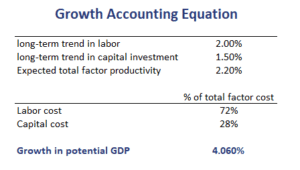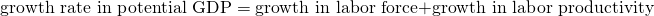# Growth Accounting Equation

We can use the Cobb-Douglas production function to estimate the growth in potential GDP. This is referred to as the growth accounting equation in macroeconomics. This methodology provides a very useful framework to estimate a reasonable growth rate for GDP. The growth rate of GDP is important because, in the long run, real stock returns are unlikely to exceed this growth rate.

On this page, we discuss the growth accounting formula, discuss a growth accounting example using Excel, and finally conclude.

## Growth accounting framework

The growth accounting equation looks as followswhere Y is the output, A is technology, K is capital, L is labor, alpha is the elasticity of output with respect to capital or the share of income paid to capital, and (1-alpha) is the elasticity of output with respect to labor or the share of income paid to labor.

## Growth account formula in practice

When applying the framework in practice, we generally will estimate the expected levels of capital and labor using their long-term trends. The shares of income going to capital (alpha) and labor (1-alpha) can be estimated using their share in the national income. The only thing that we cannot observe directly is the total factor productivity.

In practice, it is estimated as the residual. Thus, the part of total GDP growth that we cannot explain using capital growth or labor growth is attributed to technology.

## Growth accounting example

Let’s consider a growth accounting example. The following table calculates the potential growth rate for a country using inputs on the levels of capital and labor, the share in income of capital and labor, and technology## Labor productivity growth accounting equation

A second method that can be used to forecast potential GDP only uses data on the labor force and labor productivity. In particular, the labor productivity growth accounting equation focuses on changes in laborThe long-term growth rate in labor productivity reflects both capital deepening and technological progress.

## Summary

We discussed how to estimate potential growth using growth accounting relationships. In particular, we discussed two methods, the growth accounting relationship and the labor productivity growth accounting equation.